Maths-
General
Easy

Question

# The value of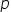for which the straight lines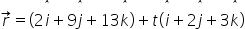and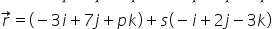are coplanar is

## -1    1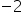2Hint:

## The correct answer is:### The given equation of the lines are as follows: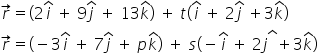We have to find the value of p such that the lines are coplanar.The conditions for coplanarity is as follows:If two lines are given by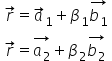Here, the first term represents the position vector of a point through which line is passing. Second term is for the vector parallel to the given line.The condition for coplanarity is as follows: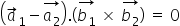If we see it is an scalar triple product. So, the scalar triple product should be zero.We will solve the scalar triple product now.So, the value of p for which the lines are coplanar is -2.

For such questions, we should know the condition for two lines to be coplanar. We should also know about the scalar triple product.#### With Turito Foundation.#### Get an Expert Advice From Turito.